Convert Digit Recognition Neural Network to Fixed Point and Generate C Code

This example shows how to convert a neural network classification model in Simulink™ to fixed point using the Fixed-Point Tool and Lookup Table Optimizer. Following the conversion, you can generate C code using Simulink Coder.

Overview

Using the Fixed-Point Tool, you can convert a design from floating point to fixed point. Use the Lookup Table Optimizer to generate memory-efficient lookup table replacements for unbounded functions such as exp and log2. Using these tools, this example shows how to convert a trained floating-point neural network classification model to use embedded-efficient fixed-point data types.

Digit Classification and MNIST Dataset

MNIST handwritten digit dataset is a commonly used dataset in the field of neural networks. For an example showing a simple way to create a two-layered neural network using this dataset, see https://blogs.mathworks.com/loren/2015/08/04/artificial-neural-networks-for-beginners/

Data and Neural Network Training

Load the data and train the network.

% Prepare Data
n = size(tr, 1);                    % number of samples in the dataset
targets  = tr(:,1);                 % 1st column is |label|
targets(targets == 0) = 10;         % use '10' to present '0'
targetsd = dummyvar(targets);       % convert label into a dummy variable
inputs = tr(:,2:end);               % the rest of columns are predictors

inputs = inputs';                   % transpose input
targets = targets';                 % transpose target
targetsd = targetsd';               % transpose dummy variable

rng(1);                             % for reproducibility
c = cvpartition(n,'Holdout',n/3);   % hold out 1/3 of the dataset

Xtrain = inputs(:, training(c));    % 2/3 of the input for training
Ytrain = targetsd(:, training(c));  % 2/3 of the target for training
Xtest = inputs(:, test(c));         % 1/3 of the input for testing
Ytest = targets(test(c));           % 1/3 of the target for testing
Ytestd = targetsd(:, test(c));      % 1/3 of the dummy variable for testing

% Train Network
hiddenLayerSize = 100;
net = patternnet(hiddenLayerSize);

[net, tr] = train(net, Xtrain, Ytrain);
view(net);

outputs = net(Xtest);
errors = gsubtract(Ytest, outputs);
performance = perform(net, Ytest, outputs);

figure, plotperform(tr);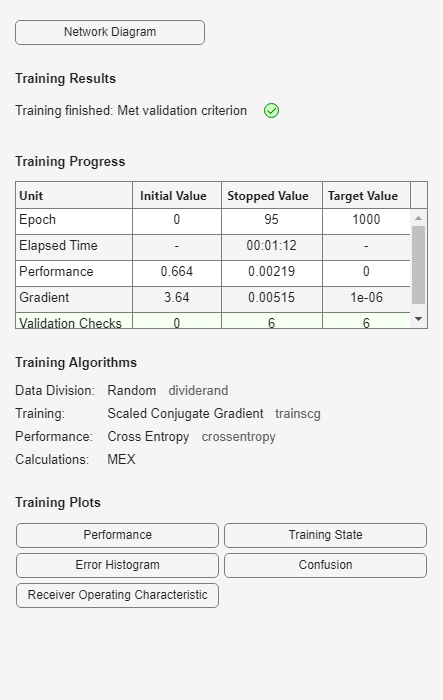Close the view of the network.

nnet.guis.closeAllViews();

Model Preparation for Fixed-Point Conversion

After training the network, use the gensim function from the Deep Learning Toolbox™ to generate a Simulink model.

sys_name = gensim(net, 'Name', 'mTrainedNN');The model generated by the gensim function contains the neural network with trained weights and biases. Prepare the trained neural network for conversion to fixed point by enabling signal logging at the output of the network, and adding input stimuli and verification blocks. The modified model is fxpdemo_mnist_classification.

Open and inspect the model.

model = 'fxpdemo_mnist_classification';
system_under_design = [model '/Pattern Recognition Neural Network'];
baseline_output = [model '/yarr'];
open_system(model);To open the Fixed-Point Tool, right-click the Function Fitting Neural Network subsystem and select Fixed-Point Tool. Alternatively, use the command-line interface of the Fixed-Point Tool. The Fixed Point Tool and its command-line interface help you prepare your model for conversion, and convert your system to fixed point. You can use the Fixed-Point Tool to collect range and overflow instrumentation of objects in your model via simulation and range analysis. In this example, use the command-line interface of the Fixed-Point Tool to convert the neural network to fixed point.

converter = DataTypeWorkflow.Converter(system_under_design);

Run Simulation to Collect Ranges

Simulate the model with instrumentation to collect ranges. Enable instrumentation using the 'Range collection using double override' shortcut. Save the simulation run name for use in later steps.

converter.applySettingsFromShortcut('Range collection using double override');
collect_ranges = converter.CurrentRunName;
sim_out = converter.simulateSystem();

Plot the correct classification rate before the conversion to establish baseline behavior.

plotConfusionMatrix(sim_out, baseline_output, system_under_design, 'Classification rate before conversion');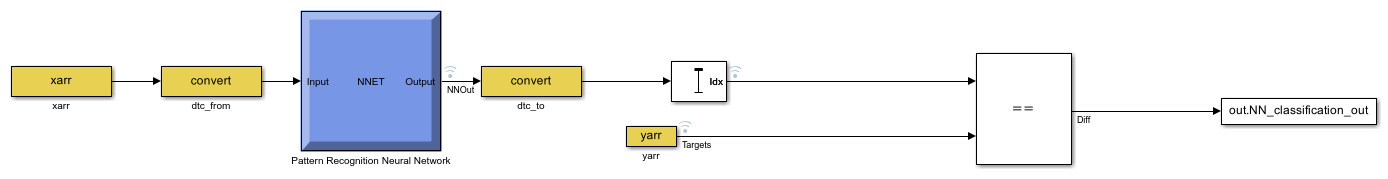Propose Fixed-Point Data Types

The Fixed-Point Tool uses range information obtained through simulation to propose fixed-point data types for blocks in the system under design. In this example, to ensure that the tools propose signed data types for all blocks in the subsystem, disable the ProposeSignedness option in the ProposalSettings object.

ps = DataTypeWorkflow.ProposalSettings;
converter.proposeDataTypes(collect_ranges, ps);

Apply Proposed Data Types

By default, the Fixed-Point Tool applies all of the proposed data types. Use the applyDataTypes method to apply the data types. If you want to only apply a subset of the proposals, in the Fixed-Point Tool use the Accept check box to specify the proposals that you want to apply.

converter.applyDataTypes(collect_ranges);

Verify Data Types

Proposed types should handle all possible inputs correctly. Set the model to simulate using the newly applied types, simulate the model, and observe that the neural network regression accuracy remains the same after the conversion.

converter.applySettingsFromShortcut('Range collection with specified data types');
sim_out = converter.simulateSystem();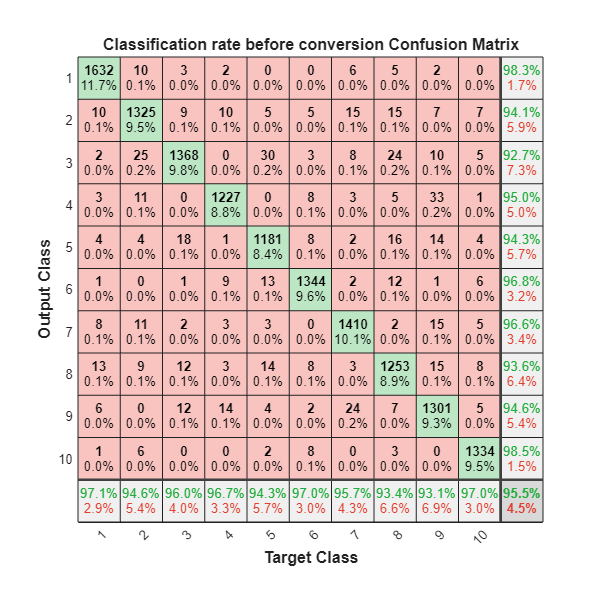Plot the correct classification rate of the fixed-point model.

plotConfusionMatrix(sim_out, baseline_output, system_under_design, 'Classification rate after fixed-point conversion');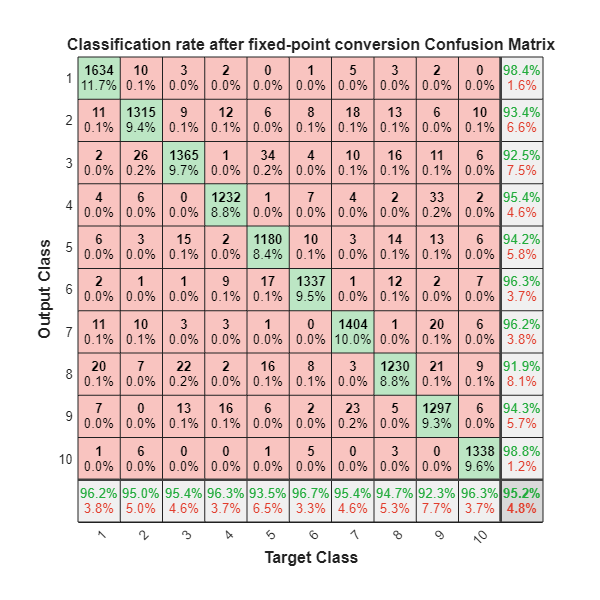Replace Activation Functions With an Optimized Lookup Table

For more efficient code, replace the Tanh Activation function in the first layer with either a lookup table or a CORDIC implementation. In this example, use the Lookup Table Optimizer to get a lookup table to replace tanh. In this example, specify EvenPow2Spacing for the breakpoint spacing for faster execution speed.

block_path = [system_under_design '/Layer 1/tansig'];
p = FunctionApproximation.Problem(block_path);
p.Options.WordLengths = 16;
p.Options.BreakpointSpecification = 'EvenPow2Spacing';
solution  = p.solve;
solution.replaceWithApproximate;
|  ID |  Memory (bits) | Feasible | Table Size | Breakpoints WLs | TableData WL | BreakpointSpecification |             Error(Max,Current) |
|   0 |             64 |        0 |          2 |              16 |           16 |         EvenPow2Spacing |     7.812500e-03, 1.000000e+00 |
|   1 |          16416 |        1 |       1024 |              16 |           16 |         EvenPow2Spacing |     7.812500e-03, 4.272461e-04 |
|   2 |           8224 |        1 |        512 |              16 |           16 |         EvenPow2Spacing |     7.812500e-03, 1.525879e-03 |

Best Solution
|  ID |  Memory (bits) | Feasible | Table Size | Breakpoints WLs | TableData WL | BreakpointSpecification |             Error(Max,Current) |
|   2 |           8224 |        1 |        512 |              16 |           16 |         EvenPow2Spacing |     7.812500e-03, 1.525879e-03 |

Follow the same steps to replace the exp function in the softmax implementation in the second layer with a lookup table.

block_path = [system_under_design '/Layer 2/softmax/Exp'];
p = FunctionApproximation.Problem(block_path);
p.Options.WordLengths = 16;
p.Options.BreakpointSpecification = 'EvenPow2Spacing';

To get an optimized lookup table, define finite lower and upper bounds for the inputs.

p.InputLowerBounds = -40;
p.InputUpperBounds = 0;
solution  = p.solve;
solution.replaceWithApproximate;
|  ID |  Memory (bits) | Feasible | Table Size | Breakpoints WLs | TableData WL | BreakpointSpecification |             Error(Max,Current) |
|   0 |             64 |        0 |          2 |              16 |           16 |         EvenPow2Spacing |     7.812500e-03, 9.996643e-01 |
|   1 |           2608 |        1 |        161 |              16 |           16 |         EvenPow2Spacing |     7.812500e-03, 6.907394e-03 |
|   2 |           1328 |        0 |         81 |              16 |           16 |         EvenPow2Spacing |     7.812500e-03, 2.451896e-02 |

Best Solution
|  ID |  Memory (bits) | Feasible | Table Size | Breakpoints WLs | TableData WL | BreakpointSpecification |             Error(Max,Current) |
|   1 |           2608 |        1 |        161 |              16 |           16 |         EvenPow2Spacing |     7.812500e-03, 6.907394e-03 |

Verify model accuracy after replacing the functions with the lookup table approximations.

converter.applySettingsFromShortcut(converter.ShortcutsForSelectedSystem{2});
sim_out = converter.simulateSystem;

plotConfusionMatrix(sim_out, baseline_output, system_under_design, 'Classification rate after function replacement');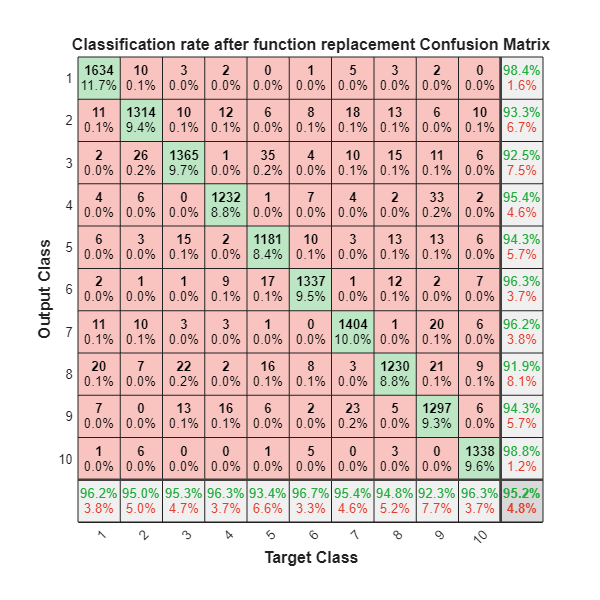Generate C code

To generate C code, right-click the Function Fitting Neural Network subsystem, select C/C++ Code > Build Subsystem. Click the Build button when prompted for tunable parameters.

Fixed-Point Designer DocumentationGet trial now